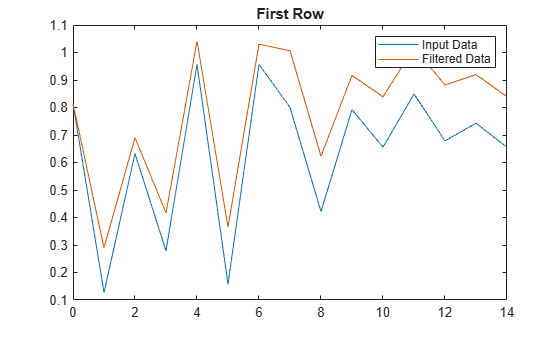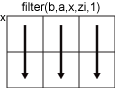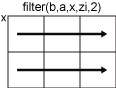# filter

1 维数字滤波器

## 语法

``y = filter(b,a,x)``
``y = filter(b,a,x,zi)``
``y = filter(b,a,x,zi,dim)``
``[y,zf] = filter(___)``

## 说明

````y = filter(b,a,x)` 使用由分子和分母系数 `b` 和 `a` 定义的有理传递函数 对输入数据 `x` 进行滤波。如果 `a(1)` 不等于 `1`，则 `filter` 按 `a(1)` 对滤波器系数进行归一化。因此，`a(1)` 必须是非零值。 如果 `x` 为向量，则 `filter` 将滤波后数据以大小与 `x` 相同的向量形式返回。如果 `x` 为矩阵，则 `filter` 沿着第一维度操作并返回每列的滤波后的数据。如果 `x` 为多维数组，则 `filter` 沿大小不等于 1 的第一个数组维度进行计算。 ```

``y = filter(b,a,x,zi)` 将初始条件 `zi` 用于滤波器延迟。`zi` 的长度必须等于 `max(length(a),length(b))-1`。`

``y = filter(b,a,x,zi,dim)` 沿维度 `dim` 进行计算。例如，如果 `x` 为矩阵，则 `filter(b,a,x,zi,2)` 返回每行滤波后的数据。`

``[y,zf] = filter(___)` 还使用任一上述语法返回滤波器延迟的最终条件 `zf`。`

## 示例

```t = linspace(-pi,pi,100); rng default %initialize random number generator x = sin(t) + 0.25*rand(size(t));```

`$y\left(n\right)=\frac{1}{windowSize}\left(x\left(n\right)+x\left(n-1\right)+...+x\left(n-\left(windowSize-1\right)\right)\right).$`

```windowSize = 5; b = (1/windowSize)*ones(1,windowSize); a = 1;```

```y = filter(b,a,x); plot(t,x) hold on plot(t,y) legend('Input Data','Filtered Data')````$H\left(z\right)=\frac{b\left(1\right)}{a\left(1\right)+a\left(2\right){z}^{-1}}=\frac{1}{1-0.2{z}^{-1}}$`

```rng default %initialize random number generator x = rand(2,15);```

```b = 1; a = [1 -0.2];```

```y = filter(b,a,x,[],2); t = 0:length(x)-1; %index vector plot(t,x(1,:)) hold on plot(t,y(1,:)) legend('Input Data','Filtered Data') title('First Row')``````figure plot(t,x(2,:)) hold on plot(t,y(2,:)) legend('Input Data','Filtered Data') title('Second Row')``````x = randn(10000,1); x1 = x(1:5000); x2 = x(5001:end);```

`$H\left(z\right)=\frac{b\left(1\right)+b\left(2\right){z}^{-1}}{a\left(1\right)+a\left(2\right){z}^{-1}}=\frac{2+3{z}^{-1}}{1+0.2{z}^{-1}}.$`

```b = [2,3]; a = [1,0.2];```

`[y1,zf] = filter(b,a,x1);`

`y2 = filter(b,a,x2,zf);`

`y1` 是来自 `x1` 的滤波后的数据，而 `y2` 是来自 `x2` 的滤波后的数据。整个滤波后的序列是 `y1``y2` 的垂直串联。

```y = filter(b,a,x); isequal(y,[y1;y2])```
```ans = logical 1 ```

## 输入参数

• 如果 `zi` 是向量，则它的长度必须是 `max(length(a),length(b))-1`

• 如果 `zi` 是矩阵或多维数组，则主维度的大小必须为 `max(length(a),length(b))-1`。剩余的每个维度的大小必须与 `x` 的相应维度的大小匹配。例如，假设沿 3×4×5 数组 `x` 的第二个维度 (`dim = 2`) 使用 `filter`。数组 `zi` 的大小必须为 [`max(length(a),length(b))-1`]×3×5。

`[]` 指定的默认值将所有滤波器延迟初始化为零。

• 如果 `dim = 1`，则 `filter(b,a,x,zi,1)``x` 的列进行运算，并返回应用于每列的过滤器。• 如果 `dim = 2`，则 `filter(b,a,x,zi,2)``x` 的行进行运算，并返回应用于每行的过滤器。## 输出参数

• 如果 `x` 是一个向量，则 `zf` 是长度为 `max(length(a),length(b))-1` 的列向量。

• 如果 `x` 是矩阵或多维数组，则 `zf` 是长度为 `max(length(a),length(b))-1` 的列向量数组，其中 `zf` 中的列数等于 `x` 中的列数。例如，假设沿 3×4×5 数组 `x` 的第二个维度 (`dim = 2`) 使用 `filter`。数组 `zf` 的大小为 [`max(length(a),length(b))-1`]×3×5。

## 详细信息

### 有理传递函数

Z 变换域中这种 `filter` 运算的输入-输出说明是一种有理传递函数。有理传递函数采用如下形式：

`$Y\left(z\right)=\frac{b\left(1\right)+b\left(2\right){z}^{-1}+...+b\left({n}_{b}+1\right){z}^{-{n}_{b}}}{1+a\left(2\right){z}^{-1}+...+a\left({n}_{a}+1\right){z}^{-{n}_{a}}}X\left(z\right),$`

，可同时处理 FIR 和 IIR 滤波器 na 是反馈滤波器阶数，nb 是前馈滤波器阶数。由于归一化，假定 a(1) = 1。

`$\begin{array}{c}a\left(1\right)y\left(n\right)=b\left(1\right)x\left(n\right)+b\left(2\right)x\left(n-1\right)+...+b\left({n}_{b}+1\right)x\left(n-{n}_{b}\right)\\ -a\left(2\right)y\left(n-1\right)-...-a\left({n}_{a}+1\right)y\left(n-{n}_{a}\right).\end{array}$``filter` 在样本 m 处的运算由时域差分方程给定

## 提示

• 要将 `filter` 函数与来自 FIR 滤波器的 `b` 系数结合使用，请使用 `y = filter(b,1,x)`

• 如果您有 Signal Processing Toolbox™，请将 `y = filter(d,x)``digitalFilter` (Signal Processing Toolbox) 对象 `d` 结合使用来对输入信号 `x` 进行滤波。要根据频率响应设定生成 `d`，请使用 `designfilt` (Signal Processing Toolbox)

• 有关滤波函数的详细信息，请参阅数字滤波 (Signal Processing Toolbox)

 Oppenheim, Alan V., Ronald W. Schafer, and John R. Buck. Discrete-Time Signal Processing. Upper Saddle River, NJ: Prentice-Hall, 1999.